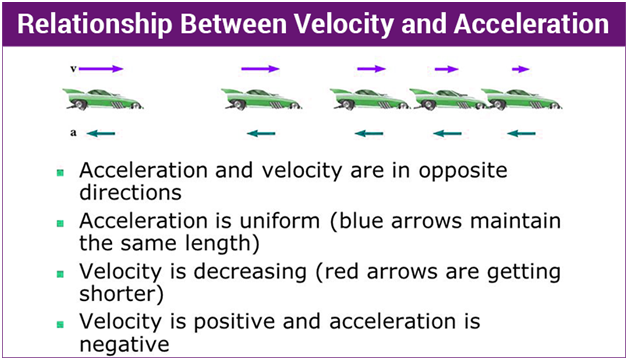# Differences Between Acceleration and Velocity

## Comparison Chart

 Comparison Basis Velocity Acceleration Meaning It alludes to the speed of an object in the given direction. Acceleration implies to any change in the velocity of an object with respect to time Calculated With Displacement Velocity What is its Nature? Vector Vector What is it? Rate of change of displacement Rate of Change of Velocity Formula Displacement/Time (d/t) Velocity/Time (v/t) Ascertains How fast an object is moving and in which direction How fast an object’s velocity changes with time. Unit of Measurement meter/second (m/s) meter/second2 (m/s2)### Acceleration:

Acceleration is a vector quantity that is defined as the rate at which an object changes its velocity. An object is accelerating if it is changing its velocity. As velocity is an example of vector, it has direction and magnitude. So we can explain the acceleration in any of these three ways:

1. a change in direction (from East to North-East)
2. a change in both speed and direction (from 34 km/h East to 12 km/h West)
3. a change in speed, the magnitude of the velocity (from 34 km/h to 67 km/h)

Below is the table of related articles explaining acceleration and velocity:

### Newton’s second law of motion:

The acceleration of an object is directly proportional to the force acting on it and inversely proportional to its mass.

Simply put, the force causes an object to accelerate, while the object’s mass resists acceleration.

### Velocity:

The amount of motion normalized to time is defined to be the velocity of the object. It is thus the displacement of the object divided by the time required to make the displacement. [The object in Tissue Tracking Echocardiography (TTE) is mainly a single point or a Region of Interest (ROI).] Hence, its unit is cm/s.

Velocity is used to describe how fast the myocardium moves in a particular direction. During systole myocardium travels towards apex and velocities are positive in nature. During diastole heart muscle moves to its initial position and diastolic velocities are negative. Displacement is the measure defining how far a myocardial segment moves from an initial point. In fetal echocardiography, its unit is mm and indicated positively.